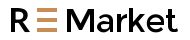Free Shipping on orders over US\$39.99

# Analyze the principle of inductive DC-DC boost of LED power supply

Inductance is a component that we use for a long time in transformer design. Its main function is to convert electrical energy into magnetic energy and then store it. It should be noted that although the structure of an Inductor is similar to a transformer, it has only one winding. This article mainly introduces the principle of inductive DC-DC booster, and this article is basic in nature, suitable for those who do not understand the characteristics of inductance, but at the same time are interested in booster friends. Some of the basic knowledge in the article can be found online, so I won’t repeat it here.

Inductance is a component that we use for a long time in transformer design. Its main function is to convert electrical energy into magnetic energy and then store it. It should be noted that although the structure of an inductor is similar to a transformer, it has only one winding. This article mainly introduces the principle of inductive DC-DC booster, and this article is basic in nature, suitable for those who do not understand the characteristics of inductance, but at the same time are interested in booster friends. Some of the basic knowledge in the article can be found online, so I won’t repeat it here.

To fully understand the principle of inductive boost, we must first know the characteristics of inductance, including electromagnetic conversion and magnetic energy storage. These two points are very important, because all the parameters we need are derived from these two characteristics.

First, let’s observe the following picture:As everyone knows, the picture above is an electromagnet, and a battery energizes a coil. Some people may be wondering, what is so easy to analyze with such a simple graph? We just want to use this simple graph to analyze what happens at the moment of power-on and power-off.

Coil (later called inductance) has a characteristic-electromagnetic conversion, electricity can be changed into magnetism, and magnetism can also be changed back to electricity. At the moment of energization, the electricity becomes magnetism and is stored in the inductor in the form of magnetism. The transient magnetism will turn into electricity and be released from the inductance when the power is off.

Now let’s look at the following picture, what happened at the moment of power failure:As I said before, the magnetic energy in the inductor will revert back to electricity when the inductor is powered off. However, the problem is that the circuit is disconnected at this time, and the current has nowhere to go. How can magnetism be converted into current? Very simple, inductance two There will be a high voltage at the end! How high is the voltage? Infinitely high, until it breaks down any medium that blocks the progress of the current.

Here we understand the second characteristic of inductance-boost characteristic. When the loop is disconnected, the energy in the inductor will be converted back into electricity in the form of infinitely high voltage. How high the voltage can rise depends only on the breakdown voltage of the medium.

Now we make a summary of the above content:

The following is a positive voltage generator, you keep flipping the switch, and you can get an infinitely high positive voltage from the input. How high the voltage rises depends on what you connect to the other end of the diode so that the current can go somewhere. If nothing is connected, the current has nowhere to go, and the voltage will rise high enough to break the switch and consume the energy in the form of heat.Then there is the negative pressure generator, you keep flipping the switch, and you can get an infinitely high negative voltage from the input.The above are all theoretical. Now let’s take a look at the actual Electronic circuit diagram to see what the smallest system of the positive/negative pressure generator looks like:

You can clearly see the evolution. In the circuit, only the switch is replaced with a triode. Don’t underestimate these two diagrams. In fact, so the switching power supply is transformed from the combination of these two diagrams, so it is very important to master these two diagrams.Finally, the issue of magnetic saturation should be mentioned. What is magnetic saturation?

Knowing from the above background, we can know that inductors can store energy and store energy in a magnetic field, but how much can it store? What happens when it is full?

1. How much to store: The parameter of maximum magnetic flux is used for this purpose. Obviously, an inductor cannot store energy indefinitely. The amount of energy it stores is determined by the product of voltage and time. For each inductor, this is a constant. With this constant, you can calculate how high an inductor must work to provide N volts and M amperes of power.

2. What happens after it is full: This is the problem of magnetic saturation. After being saturated, the inductor loses all the characteristics of electric induction, becomes a pure resistance, and consumes energy in the form of heat.